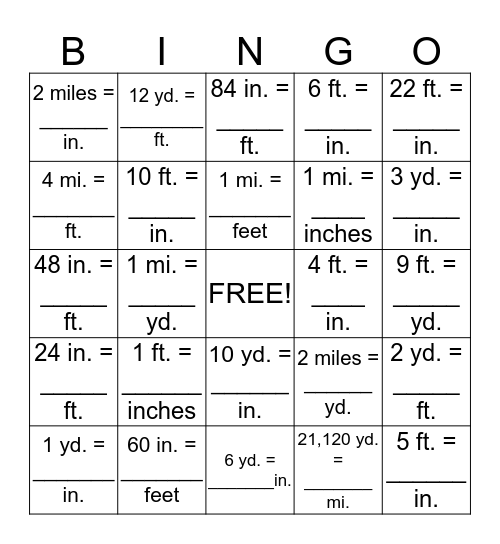# Measurement Conversion BingoThis bingo card has a free space and 24 words: 1 ft. = ______ inches, 60 in. = _______ feet, 1 mi. = _______ feet, 1 yd. = _______ in., 22 ft. = _____ in., 2 miles = ______ in., 4 mi. = _______ ft., 12 yd. = ________ ft., 4 ft. = ____ in., 10 yd. = ______ in., 1 mi. = ____ inches, 48 in. = _____ ft., 2 miles = ______ yd., 2 yd. = _____ ft., 10 ft. = _____ in., 21,120 yd. = _______ mi., 9 ft. = _____ yd., 84 in. = _____ ft., 3 yd. = _____ in., 1 mi. = _____ yd., 5 ft. = ______ in., 24 in. = _____ ft., 6 ft. = _____ in. and 6 yd. = _______in..

## Play Online